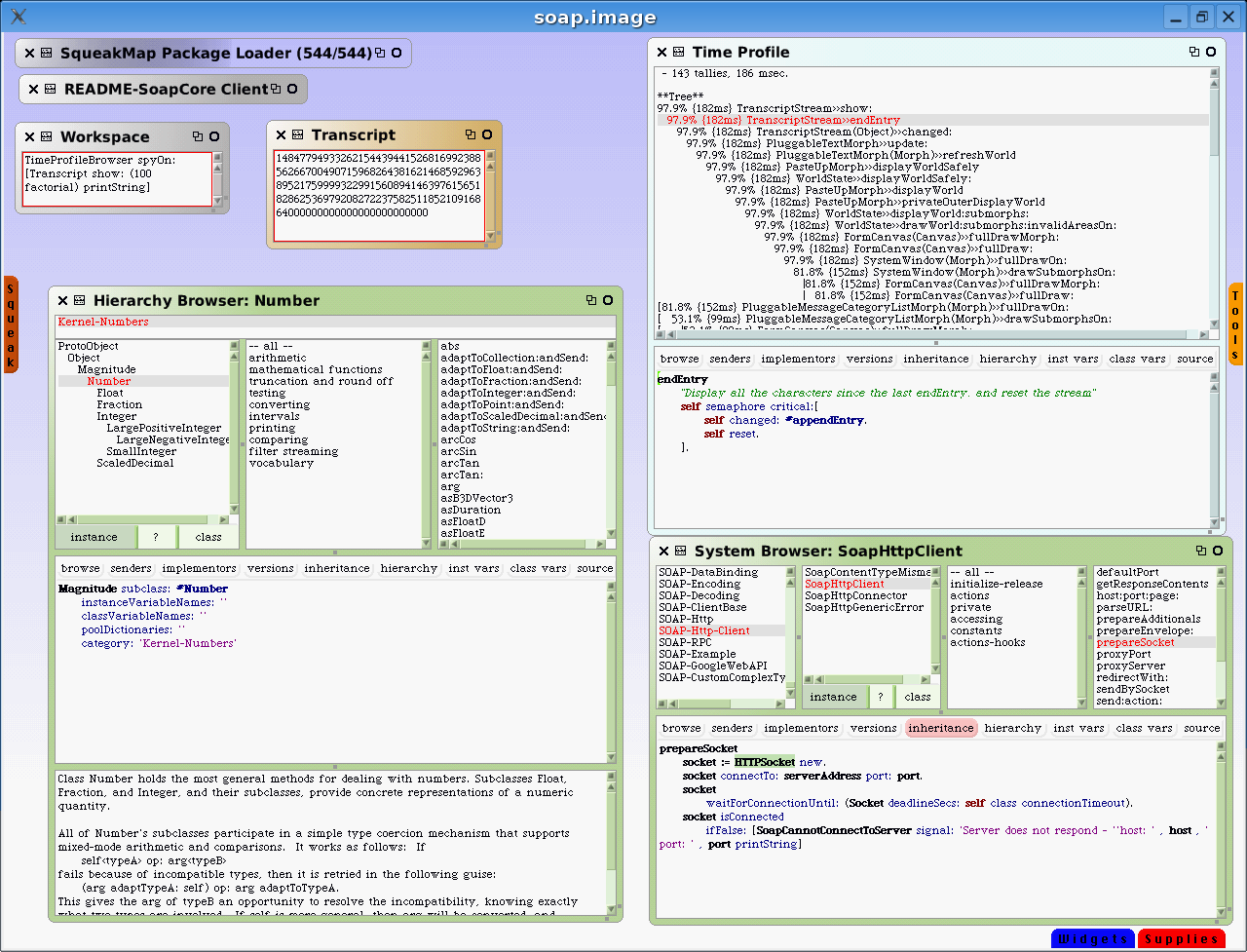# Squeak

Implementation of programming language Smalltalk

Squeak is a free MIT-licensed version of Smalltalk. It provides a fast interpreter (not JIT) written in C and Smalltalk, with ports to all common platforms and many esoteric platforms.

Squeak has a deep history going back to the origins of Smalltalk, and was developed by the original authors of Smalltalk from 1978. Modern versions of Squeak are able to compete with the best IDEs available, although the environment itself does suffer from bloat and code rot in many places.Squeak development environment

## Examples:

### Hello, World!:

Example for versions Squeak 3.10

This example would pop up an information box with “Hello, World!’ as text, and an “Okay” button.

``````self inform: 'Hello, World!'.
``````

### Fibonacci numbers:

Example for versions Squeak 3.10

This code is a method of an object, and adds n Fibonacci numbers to an OrderedCollection called `numbers`.

Variable declarations are enclosed in vertical bars and don’t specify their type. Code blocks are enclosed in square brackets. The “^” carrot at the end returns the result from this method.

``````fibonacci: n
| numbers a b |
numbers := OrderedCollection new.
a := 1.
b := 1.
[ numbers size < n ] whileTrue: [
| temp |
temp := b.
b := a + b.
a := temp.
].
^ numbers.
``````

### Fibonacci numbers:

Example for versions Squeak 3.10

This is a recursive method implementing the Fibonacci sequence, writing it to the given stream of numbers.

To invoke this method:

stream := WriteStream on: (Array new: 100). Fibonacci new fibonacci: 1 and: 1 writeTo: stream

There is no halting condition; the program will continue writing Fibonacci numbers until interrupted.

This example is far from optimal: the stream will automatically double the size of the array each time it is filled (a very expensive operation in Squeak), and the recursion continually consumes memory. The alternative iterative example is much more efficient.

``````fibonacci: n1 and: n2 writeTo: stream
stream nextPut: n1.
self fibonacci: n2 and: (n1+n2) writeTo: stream.
``````

### Factorial:

Example for versions Squeak 3.10

This example is actually cut and paste from the Squeak core source code in the Integer class. From any Squeak image:

10000 factorial. “and press alt-p to print the result below this line”

284625968091705451890641321211986889014805140170279923079417999427441134000376444377299078675778477581588406214231752883004233994015351873905242116138271617481982419982759241828925978789812425312059465996259867065601615720360323979263287367170557419759620994797203461536981…etc… edited for brevity.

This takes about 200 milliseconds on a modern PC to compute the factorial, and about 6 seconds to convert the resulting number into a String for displaying.

The resulting number has this 35660 digits:

10000 factorial asString size

35660

``````factorial

self = 0 ifTrue: [^ 1].
self > 0 ifTrue: [^ self * (self - 1) factorial].
self error: 'Not valid for negative integers'
``````